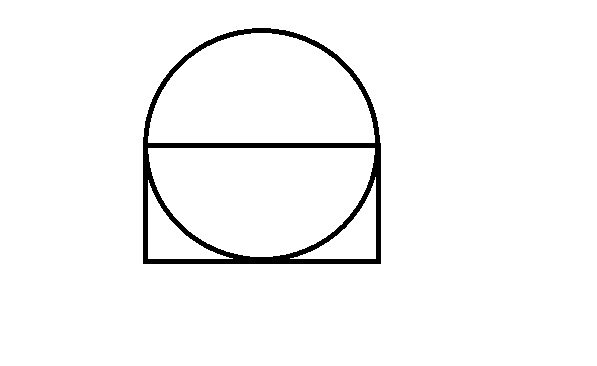# Mensuration Questions for RRB NTPC Set-3 PDF

0
663

## Mensuration Questions for RRB NTPC Set-3 PDF

Download RRB NTPC Mensuration Questions Set-3 PDF. Top 10 RRB NTPC questions based on asked questions in previous exam papers very important for the Railway NTPC exam.

Question 1: The height of a triangle is equal to the perimeter of a square whose diagonal is 14.14m and the base of the same triangle is equal to the side of the square whose area is 784 m2. What is the area of the triangle? (in m2)

a) 504

b) 560

c) 478

d) 522

e) 496

Question 2: The diameter of a wheel is 49 m. How many revolutions will it make to cover a distance of 3200 m?

a) 17

b) 27

c) 24

d) 21

e) 18

Question 3: The area of a rectangle is equal to the area of a circle with circumference equal to 220 m What is the length of the rectangle of its breadth is 50 m ?

a) 56 m

b) 83 m

c) 77 m

d) 69 m

e) None of these

Question 4: The perimeter of a square is one-fourth the perimeter of a rectangle If the perimeter of the square is 44 cm and the length of the rectangle is 51 cm what is the difference between the breadth of the rectangle and the side of the square ?

a) 30 cm

b) 18 cm

c) 26 cm

d) 32 cm

e) None of these

Question 5: What would be the area of a square whose diagonal measures 28 cm?

a) 288 $cm^{2}$

b) 514 $cm^{2}$

c) 428 $cm^{2}$

d) 392 $cm^{2}$

e) None of these

Question 6: The circumference of a circle is 748 metres. What will be its radius ?

a) 113 metres

b) 107 metres

c) 116 metres

d) 109 metres

e) None of these

Question 7: If the area of the rectangle is 1248 square metres and its breadth is 32 metres, what is its perimeter ?

a) 142 metres

b) 128 metres

c) 148 metres

d) 124 metres

e) None of these

Question 8: If the perimeter of a rectangle is 138 metres and the difference between the length and breadth is 7 metres, what is the area of the rectangle ?

a) 1216 square metres

b) 1147 square metres

c) 1184 square metres

d) 1178 square metres

e) None of these

Question 9: The area of a circle is 2464 square metres. What will its circumference be ?

a) 132 metres

b) 176 metres

c) 231 metres

d) 272 metres

e) 109 metres

Question 10: Area of the circle is 616 $cm^{2}$. What is the area of the rectangle? (One side of the rectangle passes through the centre of the circle)a) 786 $cm^{2}$

b) 196 $cm^{2}$

c) 392 $cm^{2}$

d) Cannot be determined

e) None of these

We know that, diagonal = 1.414* side
Here, diagoal = 14.14, side = 10 m
Height = Perimeter of square = 10*4 = 40m
Now, base = side of square with 784 $m^2$ area.
base = 28
Area of triangle = .5*28*40 = 560 $m^2$

Option B is correct option.

Circumference of th wheel = pi * Diameter = (22/7) * 49 = 154
Number of revolutions of the wheel = 3200/154 = 20.77~21
The wheel will make around 21 revolutions.

Ciircumference = 2*(22/7)*r = 220
Hence, r = 35
Now, area of circle = (22/7)*35*35 = 3850
Area of rectangle is same as that of circle
Area of rectangle = 3850
length = 3850/50 = 77m
Therefore, option C is correct answer.

Perimeter of rectangle = 4(44) = 176 cm
Difference between side of square and breadth of rectangle = 37 – 11 = 26 cm
Therefore, the correct option is option C.

We know that,
Diagonal = side * 1.414
Therefore, side = 28/1.414 = 19.8
Area of square = 19.8 *19.8 = 392.04 ~ 392
Therefore, the correct option is option D.

As we know that circumference of  a circle will be = $2 \pi r = 2 \times \frac{22}{7} \times r$ (Where r is the radius of the circle)

Now parameter is given as 748 m.

Hence, its radius will be = $748 \times 7 \div 44$ = 119

As we know that the area of a rectangle is $l \times b$ (Where l is length of rectangle and b is breadth of rectangle)

Which is 1248 square meter with breadth(b) 32 meters

So length will be = $\frac{1248}{32} = 39$

Hence, parameter will be equal to = 2(39+32) = 142 m

Let the length be x and breadth be y.
x – y = 7
The perimeter of rectangle is 138
2x + 2y = 138
x + y = 69

2x = 76
x = 38
y = 31

Area of rectangle = 31*38 = 1178
Option D is correct.

Area of circle = (22/7)*r*r
r^2 = (7/22)*2464
r^2 = 784
r=28
Circumference = 2*(22/7)*28  =176 metre
Option B is correct.
Area of the circle =$\pi d^2/4$ = 616